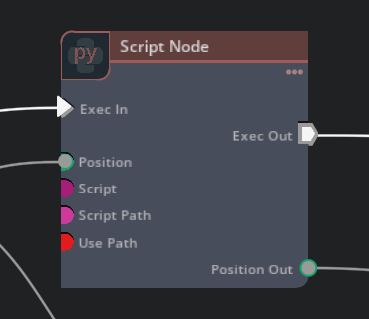# AutoFunc functionality with Replicator

Hello,
I am getting an error when trying to run the AutoFunc camera example
copied below. The error message is :

`[Error] [omni.graph.core.plugin] Can not connect /Replicator/SDGPipeline/ComputeCameraPos/outputs_out_0 to /Replicator/SDGPipeline/OgnWritePrimAttribute.inputs:values: bundles can only connect to other bundles.`

I am using Omniverse Code 2022.3.1 with Kit 104.1

``````import omni.replicator.core as rep
import omni.graph.core as og
import numpy as np

with rep.new_layer():

# Randomize the camera position using AutoFunc
frame = 0

@og.AutoFunc(module_name="omni.replicator")
def ComputeCameraPos(distance: float, elevation: float, numSamples: int = 1) -> og.Bundle:
# Note 1: numSamples input currently required
# Note 2: Only bundle output currently supported, this will be expanded in the future.

global frame
print("FRAME", frame)
frame += 1
x = np.cos(azimuth) * np.cos(elevation) * distance
y = np.sin(azimuth) * np.cos(elevation) * distance
z = np.sin(elevation) * distance

bundle = og.Bundle("return", False)
bundle.create_attribute("values", og.Type(og.BaseDataType.DOUBLE, 3, 1)).value = [[x, y, z]]
return bundle

# This will allow the AutoFunc return attribute `out_0` to be automatically connected to the pose node's `values` input

# Register functionality into replicator
def camera_randomizer(distance: float, elevation: float):
return rep.utils.create_node("omni.replicator.ComputeCameraPos", distance=distance, elevation=elevation)

# Register randomizer
rep.randomizer.register(camera_randomizer)

# TEST
camera = rep.create.camera()
render_product = rep.create.render_product(camera, (1024, 1024))

rep.create.cube()

# Initialize and attach writer
writer = rep.WriterRegistry.get("BasicWriter")
writer.initialize(output_dir="_output_camera_function", rgb=True, bounding_box_2d_tight=True)
writer.attach([render_product])

with rep.trigger.on_frame(num_frames=100):
with camera:
rep.modify.pose(
position=rep.randomizer.camera_randomizer(400, 45),
look_at=(0,0,0)
)
``````

Hello @engineer_a1! I’ve shared your post with the dev team for further assistance.

1 Like

I can confirm autofunc is not working in current versions of Code. Autofunc is a bit on an experimental feature that’s in active development. I know this might not be ideal, but the two current work arounds are:

1. You can write a custom ogn node and reference it in the rep scripts to execute arbitrary python.
2. You can also trigger an Action Graph in the stage, through a custom event via rep.

Something like this:

``````import omni.replicator.core as rep
import omni.graph.core as og

with rep.new_layer():

# Assign the camera, Init the Writer to output RGB, and the output directory
render_product = rep.create.render_product('/World/Camera', (2048, 1536))
writer = rep.WriterRegistry.get("BasicWriter")
writer.initialize( output_dir="_Test", rgb=True)
writer.attach([render_product])

# This links the Action Script to replicator
# This will trigger an event in the Action Script named 'foo'
# You have to create the action script, with the event in order for this to work.
trigger = rep.trigger.on_frame(num_frames=10)
publish_event_node = og.Controller().create_node('/Replicator/SDGPipeline/MyTest', 'omni.graph.action.SendCustomEvent')
publish_event_node.get_attribute('inputs:eventName').set('foo')
trigger.node.get_attribute("outputs:execOut").connect(publish_event_node.get_attribute("inputs:execIn"), True)
``````

This rep script wires a trigger custom event node into the graph that gets generated. When the rep graph runs, it triggers the event in your custom action graph, where you can perform arbitrary logic.

Within your action graph, you can run logic as omnigraph nodes, or you can use python wrapped within a script node: